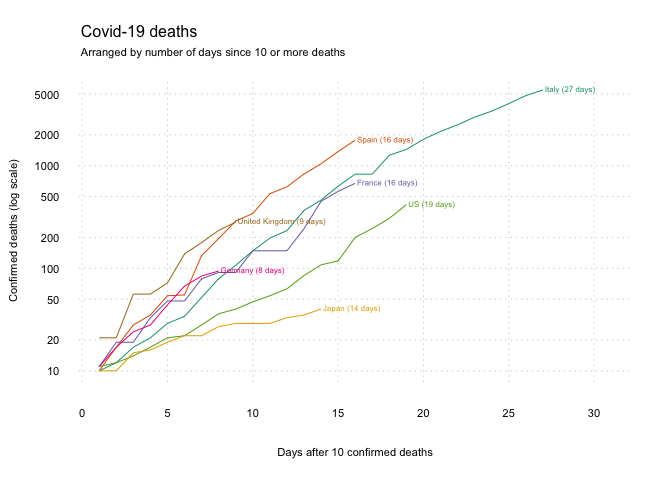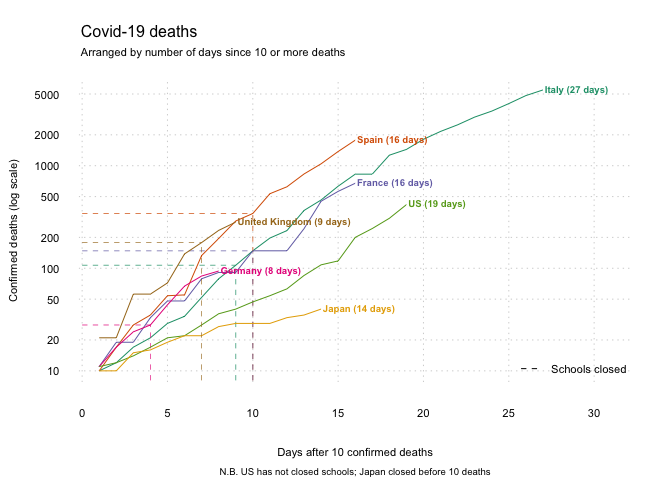In the previous post I showed how to use data from Johns Hokins to create a graph of the deaths from Covid-19.

In this post, I’ll show how we can use data from UNESCO to mark the dates when schools closed.

First, we need to run the previous .R script and use read.csv() to load the data.

# Run the previous .R script
source('COVID_DEATHS_GRAPHS.R')# Load data
data_raw <- read.csv('https://en.unesco.org/sites/default/files/covid_impact_education.csv', as.is = TRUE)

As before, there are various data-management steps. This time we need to change the names of the UK and the US to match the previous dataset, and only keep the countries we want to use.

# Re-name UK and US
data_raw$Country[data_raw$Country=='United Kingdom of Great Britain and Northern Ireland'] <- 'United Kingdom'data_raw$Country[data_raw$Country=='United States of America'] <- 'US'

# Keep only the selected countries
schools <- data_raw[data_raw$Country %in% countries & data_raw$Scale == 'National', ]

We can now add the data to the plot from before. The code from the previous plot is repeated below, with an additional few steps in the for() loop that produced the trend lines.

# Start with an 'empty' plot of Italy
plot_data <- data[data$Group.1=='Italy', ]plot(plot_data$x, type = 'n',
log = 'y', # y-axis is on the log scale
bty = 'n', # no border around the plot
xlim = c(1,(nrow(plot_data)+4)),
axes = FALSE, bg='gray80',
xlab='Days after 10 confirmed deaths',
ylab='Confirmed deaths (log scale)',
sub = 'N.B. US has not closed schools; Japan closed before 10 deaths',
cex.sub=.6,
cex.lab=.7)

grid(nx = NULL, ny = NULL, col = "lightgray", lty = "dotted", lwd = par("lwd"), equilogs = F)

# Add each of the other countries
for (country in countries){
plot_data <- data[data$Group.1 == country,] # Plot the lines lines(c(1:nrow(plot_data)), plot_data$x,
col=cols[which(countries==country)])
# Skip the US, because schools haven't closed
if (country != 'US'){
# Create marker for when schools first closed
closed <- schools[schools$Country==country,] closed <- data.frame(date = as.Date(closed[1,1], '%d/%m/%Y'), now=1) # Merge the marker with the plot data plot_data_s <- merge(plot_data, closed, by.x='Group.2', by.y='date', all.x=T) # Identify day and deaths when schools closed closed <- c(which(plot_data_s$now==1),
plot_data_s$x[which(plot_data_s$now==1)])

lines(x=c(closed,closed),
y=c(5, closed),
col=cols[which(countries==country)],
lty=2, lwd=.7)
lines(x=c(0,closed),
y=c(closed, closed),
col=cols[which(countries==country)],
lty=2, lwd=.7)
}
text(x=nrow(plot_data), y=max(plot_data\$x),
label=paste0(country,' (',nrow(plot_data),' days)'),
pos=4, offset=.1, cex=.6, font=2,
col=cols[which(countries==country)])
}

axis(1, lwd=0, cex.axis=.7)
axis(2, lwd=0, las=1, cex.axis=.7)

bty='n', cex=.7)